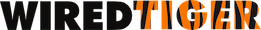Version 10.0.2
Join cursors

Join cursors provide a way to iterate over a subset of a table, where the subset is specified by relationships with reference cursors.

A join cursor is created with WT_SESSION::open_cursor using a `"join:table:<name>"` URI prefix. Then reference cursors are positioned to keys on indices and joined to the join cursor using WT_SESSION::join calls. The result is a join cursor that can be iterated to satisfy the join equation.

Here is an example using join cursors:

/* Open cursors needed by the join. */
error_check(session->open_cursor(session, "join:table:poptable", NULL, NULL, &join_cursor));
error_check(
session->open_cursor(session, "index:poptable:country", NULL, NULL, &country_cursor));
error_check(
session->open_cursor(session, "index:poptable:immutable_year", NULL, NULL, &year_cursor));
/* select values WHERE country == "AU" AND year > 1900 */
country_cursor->set_key(country_cursor, "AU\0\0\0");
error_check(country_cursor->search(country_cursor));
error_check(session->join(session, join_cursor, country_cursor, "compare=eq,count=10"));
year_cursor->set_key(year_cursor, (uint16_t)1900);
error_check(year_cursor->search(year_cursor));
error_check(
session->join(session, join_cursor, year_cursor, "compare=gt,count=10,strategy=bloom"));
/* List the values that are joined */
while ((ret = join_cursor->next(join_cursor)) == 0) {
error_check(join_cursor->get_key(join_cursor, &recno));
error_check(join_cursor->get_value(join_cursor, &country, &year, &population));
printf("ID %" PRIu64, recno);
printf(
": country %s, year %" PRIu16 ", population %" PRIu64 "\n", country, year, population);
}
scan_end_check(ret == WT_NOTFOUND);

Joins support various comparison operators: `"eq"`, `"gt"`, `"ge"`, `"lt"`, `"le"`. Ranges with lower and upper bounds can also be specified, by joining two cursors on the same index, for example, one with `"compare=ge"` and another `"compare=lt"`. In addition to joining indices, the main table can be joined so that a range of primary keys can be specified.

By default, a join cursor returns a conjunction, that is, all keys that satisfy all the joined comparisons. By specifying a configuration with `"operation=or"`, a join cursor will return a disjunction, or all keys that satisfy at least one of the joined comparisons. More complex joins can be composed by specifying another join cursor as the reference cursor in a join call.

Here is an example using these concepts to show a conjunction of a disjunction:

/* Open cursors needed by the join. */
error_check(session->open_cursor(session, "join:table:poptable", NULL, NULL, &join_cursor));
error_check(session->open_cursor(session, "join:table:poptable", NULL, NULL, &subjoin_cursor));
error_check(
session->open_cursor(session, "index:poptable:country", NULL, NULL, &country_cursor));
error_check(
session->open_cursor(session, "index:poptable:country", NULL, NULL, &country_cursor2));
error_check(
session->open_cursor(session, "index:poptable:immutable_year", NULL, NULL, &year_cursor));
/*
* select values WHERE (country == "AU" OR country == "UK")
* AND year > 1900
*
* First, set up the join representing the country clause.
*/
country_cursor->set_key(country_cursor, "AU\0\0\0");
error_check(country_cursor->search(country_cursor));
error_check(
session->join(session, subjoin_cursor, country_cursor, "operation=or,compare=eq,count=10"));
country_cursor2->set_key(country_cursor2, "UK\0\0\0");
error_check(country_cursor2->search(country_cursor2));
error_check(
session->join(session, subjoin_cursor, country_cursor2, "operation=or,compare=eq,count=10"));
/* Join that to the top join, and add the year clause */
error_check(session->join(session, join_cursor, subjoin_cursor, NULL));
year_cursor->set_key(year_cursor, (uint16_t)1900);
error_check(year_cursor->search(year_cursor));
error_check(
session->join(session, join_cursor, year_cursor, "compare=gt,count=10,strategy=bloom"));
/* List the values that are joined */
while ((ret = join_cursor->next(join_cursor)) == 0) {
error_check(join_cursor->get_key(join_cursor, &recno));
error_check(join_cursor->get_value(join_cursor, &country, &year, &population));
printf("ID %" PRIu64, recno);
printf(
": country %s, year %" PRIu16 ", population %" PRIu64 "\n", country, year, population);
}
scan_end_check(ret == WT_NOTFOUND);

All the joins should be done on the join cursor before WT_CURSOR::next is called. Calling WT_CURSOR::next on a join cursor for the first time populates any bloom filters and performs other initialization. The join cursor's key is the primary key (the key for the main table), and its value is the entire set of values of the main table. A join cursor can be created with a projection by appending `"(col1,col2,...)"` to the URI if a different set of values is needed.

Keys returned from the join cursor are ordered according to the first reference cursor joined. For example, if an index cursor was joined first, that index determines the order of results. If the join cursor uses disjunctions, then the ordering of all joins determines the order. The first join in a conjunctive join, or all joins in a disjunctive join, are distinctive in that they are iterated internally as the cursor join returns values in order. Any bloom filters specified on the joins that are used for iteration are not useful, and are silently ignored.

When disjunctions are used where the sets of keys overlap on these 'iteration joins', a join cursor will return duplicates. A join cursor never returns duplicates unless `"operation=or"` is used in a join configuration, or unless the first joined cursor is itself a join cursor that would return duplicates.

Another example of using a join cursor is provided in ex_col_store.c. Here the columns hour and temp are joined together to find the maximum and minimum temperature for a given time period.

/* Open cursors needed by the join. */
error_check(
session->open_cursor(session, "join:table:weather(hour,temp)", NULL, NULL, &join_cursor));
error_check(
session->open_cursor(session, "index:weather:hour", NULL, NULL, &start_time_cursor));
error_check(session->open_cursor(session, "index:weather:hour", NULL, NULL, &end_time_cursor));
/*
* Select values WHERE (hour >= start AND hour <= end). Find the starting record closest to
* desired start time.
*/
start_time_cursor->set_key(start_time_cursor, start_time);
error_check(start_time_cursor->search_near(start_time_cursor, &exact));
if (exact == -1) {
ret = start_time_cursor->next(start_time_cursor);
if (ret == WT_NOTFOUND)
return ret;
else
error_check(ret);
}
error_check(session->join(session, join_cursor, start_time_cursor, "compare=ge"));
/* Find the ending record closest to desired end time. */
end_time_cursor->set_key(end_time_cursor, end_time);
error_check(end_time_cursor->search_near(end_time_cursor, &exact));
if (exact == 1) {
ret = end_time_cursor->prev(end_time_cursor);
if (ret == WT_NOTFOUND)
return ret;
else
error_check(ret);
}
error_check(session->join(session, join_cursor, end_time_cursor, "compare=le"));
/* Initialize minimum temperature and maximum temperature to temperature of the first record. */
ret = join_cursor->next(join_cursor);
if (ret == WT_NOTFOUND)
return ret;
else
error_check(ret);
error_check(join_cursor->get_key(join_cursor, &recno));
error_check(join_cursor->get_value(join_cursor, &hour, &temp));
*min_temp = temp;
*max_temp = temp;
/* Iterating through found records between start and end time to find the min & max temps. */
while ((ret = join_cursor->next(join_cursor)) == 0) {
error_check(join_cursor->get_value(join_cursor, &hour, &temp));
*min_temp = WT_MIN(*min_temp, temp);
*max_temp = WT_MAX(*max_temp, temp);
}
WT_SESSION::open_cursor
int open_cursor(WT_SESSION *session, const char *uri, WT_HANDLE_NULLABLE(WT_CURSOR) *to_dup, const char *config, WT_CURSOR **cursorp)
Open a new cursor on a data source or duplicate an existing cursor.
WT_SESSION::join
int join(WT_SESSION *session, WT_CURSOR *join_cursor, WT_CURSOR *ref_cursor, const char *config)
Join a join cursor with a reference cursor.
WT_NOTFOUND
#define WT_NOTFOUND